﻿ 城市防汛移动电源排水泵车-上海黄河泵业制造有限公司一官方网站

021-60259216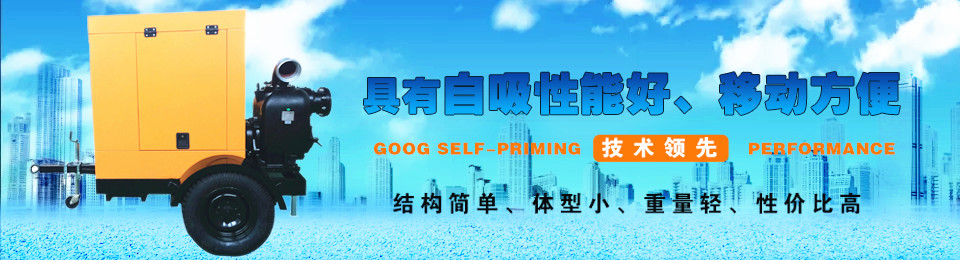#### 城市防汛移动电源排水泵车

1.每台泵车是一个独立的移动泵站，无需任何泵站工程； 2.机动性作业，随时到达；高排水量或远距离输送； 3.单人短时间内安装操作；不需当地电源和起吊设备；无复杂维护保养。

随着文明城市建设脚步的加快，城市对各个层面的应急预案已愈加完善。由于应急排涝抢险与常规的养护作业有许多特殊性， 如：事件的突发性，抢险的紧迫性，工作面的复杂性，作业的安全性，施工环境的随机性，工作场地的环保性，时间的任意性，连续工作时间长……这就对相应的设备也提出了更高的，更专业性的要求。

该车机动性强，真正实现了移动水泵的概念，设备可满足紧急排水抢险的需要，并具备移动电源功能；配备液压支腿，保证施工作业时整车稳定性；设备能够正常可靠工作，有良好的通风和散热功能；整车涉水深度为600mm，可满足深水区域抢险救援工作；具有电源输出功能，可满足230/400V用电需要；照明可以满足夜间操作的需要和安全性。
本装备移动灵活、展开和撤收速度快、投入作业人员少、适应性强、劳动强度低、应急反应速度快，提高了城市救灾应急的综合保障性能。

1.无固定泵站及无电源地区；
2. 突击防洪排涝；
3.抗旱抢险；
4.农业灌溉、水产养殖；
5.各种临时抽水场合；
6. 围堰抽水；
7.工业、市政排水。

排水量300-3500立方/小时，扬程5-200米，功率30-350KW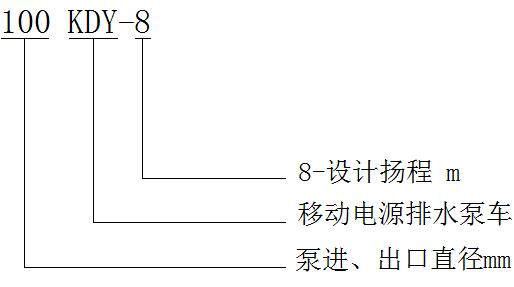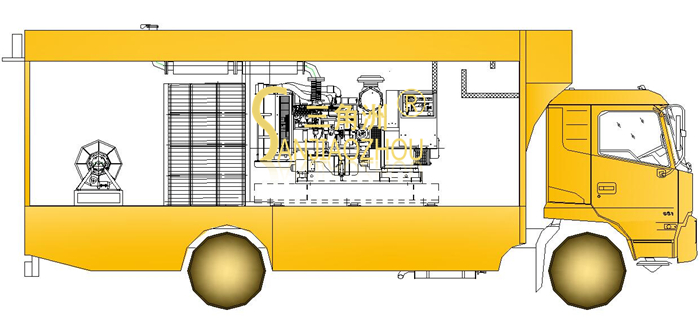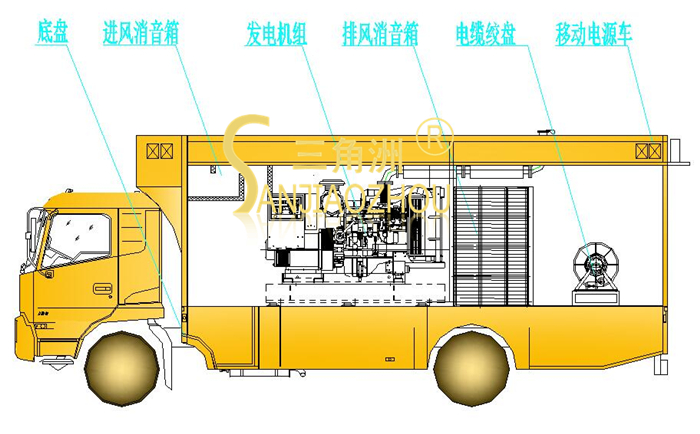型  号    流量（m3/h）   扬程(m)     转速          功率(KW) 100KDY-8  Q=90m3/h   H=8m    n=2900rpm  N(配用）4HP/3KW 150KDY-5  Q=180m3/h   H=5m    n=1450rpm   N(配用)5HP/4KW 150KDY-5  Q=230m3/h   H=8.2m   n=1850rpm  N(配用)12HP/7.5KW 200KDY-8  Q=360m3/h   H=8m   n=1450rpm  N(配用)15HP/11KW 200KDY-8  Q=340m3/h   H=7.1m   n=1360rpm  N(配用)12HP/11KW 200KDY-4  Q=340m3/h   H=4m   n=1060rpm  N(配用)8HP/5.5KW 200KDY-10  Q=450m3/h   H=10m   n=1450rpm  N(配用)30HP/18.5KW 200KDY-12  Q=500m3/h   H=12.2m  n=1600rpm  N(配用)40HP/30KW 250KDY-4  Q=450m3/h   H=4.8m  n=980rpm   N(配用)12HP/11KW 250KDY-12  Q=650m3/h   H=11.6m  n=1450rpm  N(配用)40HP/30KW 250KDY-14  Q=720m3/h   H=14.3m  n=1600rpm  N(配用)60HP/37KW 250KDY-7  Q=450m3/h   H=7m   n=980rpm   N(配用)20HP/15KW 250KDY-5  Q=400m3/h   H=5.5m  n=870rpm   N(配用)12HP/11KW 250KDY-8  Q=540m3/h   H=8m   n=1100rpm  N(配用)25HP/18.5KW 300KDY-7  Q=780m3/h   H=7m   n=980rpm   N(配用)30HP/22KW 300KDY-7  Q=585m3/h   H=3.9m  n=730rpm   N(配用)12HP/11KW 300KDY-7  Q=1035m3/h   H=12.3m  n=1300rpm  N(配用)80HP/55KW 300KDY-4  Q=710m3/h   H=4m   n=780rpm   N(配用)15HP/11KW 350KDY-8  Q=1000m3/h   H=8m   n=980rpm   N(配用)40HP/30KW 350KDY-8  Q=745m3/h   H=4.4m  n=730rpm   N(配用)20HP/15KW 350KDY-4  Q=1000m3/h   H=4.3m  n=980rpm   N(配用)30HP/22KW 400KDY-7  Q=1260m3/h   H=6.8m  n=730rpm   N(配用)40HP/30KW 400KDY-7  Q=1692m3/h   H=12.3m  n=980rpm   N(配用)100HP/75KW 400KDY-10  Q=1400m3/h   H=10m   n=730rpm   N(配用)66HP/55KW 400KDY-10  Q=1880m3/h   H=18m   n=980rpm   N(配用)165HP/110KW 500KDY-6  Q=1980m3/h   H=6.2m  n=580rpm   N(配用)60HP/55KW 500KDY-10  Q=2492m3/h   H=9.8m  n=730rpm   N(配用)120HP/95KW 650KDY-7  Q=3550m3/h   H=7.1m  n=470rpm   N(配用)135HP/100KW 650KDY-7  Q=3400m3/h   H=6.5m  n=450rpm   N(配用)120HP/90KW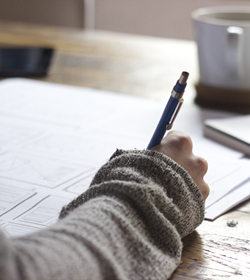1、量身定制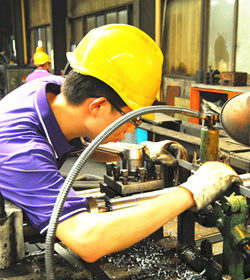2、专业生产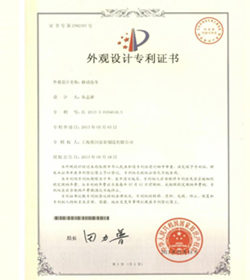3、专利保障4、服务周到

## 黄河泵业定制流程

• 01

提供资料

• 02

黄河设计

• 03

方案确定

• 04

制作水利模型

• 05

生产成型

• 06

性能测试

• 07

合格出厂

• 08

客户验收联系黄河 / CONTACT AOKAI